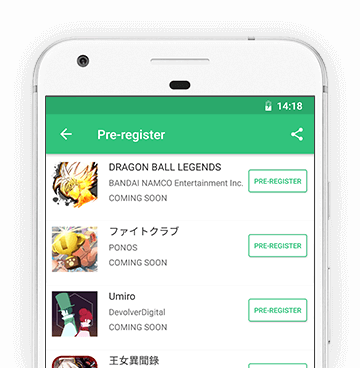We use cookies and other technologies on this website to enhance your user experience.# 浮島物語～最強刻印士になるストーリー～

for Android

## The description of 浮島物語～最強刻印士になるストーリー～

アメイジングで不思議な異世界でアドベンチャーを楽しめる！

=·=·=·=·=·=·=·=·=·=·=·=·=·=·=·=·=·=·=·=·=·=·=·=·=·=·=·=·=·=·=·=·=·=·=··=·=·=·=·=·=·=·
◆世界観

そんな世界で、最強の王者が記憶を喪失したらどうなる？

◆ハイクオリティー美人キャラクター

◆放置材料で鍛冶、手作、調理、錬成

◆共同戦闘

=·=·=·=·=·=·=·=·=·=·=·=·=·=·=·=·=·=·=·=·=·=·=·=·=·=·=·=·=·=·=·=·=·=·=··=·=·=·=·=·=·=·
◆声優
■ 植田佳奈
■ 柚木涼香
■ 小清水亜美
■ 斎藤千和
■ 中原麻衣
■ 小澤亜李
■ 名塚佳織
■ 井上麻里奈
■ 小松未可子
■ 佐藤利奈
■ 日笠陽子
■ 田中理恵

◆イラストレーター
■　　ギザン
■ スコッティ
■ ハヤブサ
■ 八城惺架
■ スカイ
■ ましゅー
---------------------------------------------

## Pre-Register Details

Pre-Registrants: 106

Release Date: COMING SOON

## 浮島物語～最強刻印士になるストーリー～ Tags